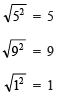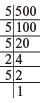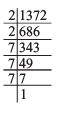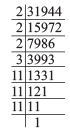Courses

# Points to Remember- Cubes and Cube Roots Class 8 Notes | EduRev

## Mathematics (Maths) Class 8

Created by: Full Circle

## Class 8 : Points to Remember- Cubes and Cube Roots Class 8 Notes | EduRev

The document Points to Remember- Cubes and Cube Roots Class 8 Notes | EduRev is a part of the Class 8 Course Mathematics (Maths) Class 8.
All you need of Class 8 at this link: Class 8

Facts That Matter

• If we multiply a number by itself three times, the product so obtained is called the perfect cube of that number.
• There are only 10 perfect cubes from 1 to 1000.
• Cubes of even numbers are even and those of odd numbers are odd.
• The cube of a negative number is always negative.
• If the digit in the one’s place of a number is 0, 1, 4, 5, 6 or 9, then its cube will end in the same digit.
• If the digit in the one’s place of a number is 2, then the ending digit of its cube will be 8 and vice-versa.
• If the digit in the one’s place of a number is 3, then the ending digit of its cube will be 7 and vice-versa.
• If the prime factors of a number cannot be made into groups of 3, it is not a perfect cube.
• The symbol ∛ denotes the cube root of a number, such as ∛64 = 4.

We Know That
The square of a number is obtained by multiplying the number by itself, i.e.
2 * 2 = 22
4 * 4 = 42
5 * 5 = 52
Finding square root is the inverse operation of finding the square of a number, i.e.A natural number multiplied by itself three times gives cube of that number, e.g.
1 * 1 * 1 = 1
2 * 2 * 2 = 8
3 * 3 * 3 = 27
4 * 4 * 4 = 64
The numbers 1, 8, 27, 64, … are called cube numbers or perfect cubes.

Properties of Perfect Cubes
Property I: If the digit in the one’s place of a number is 0, 1, 4, 5, 6 or 9, then the digit in the one’s place of its cube will also be the same digit.
Property II: If the digit in the one’s place of a number is 2, the digit in the one’s place of its cube is 8, and vice-versa.
Property III: If the digit in the one’s place of a number is 3, the digit in the one’s place of its cube is 7 and vice-versa.
Property IV: Cubes of even natural numbers are even.
Examples:

 Number Cube Number Cube 836 51246656 1218 17285832

Property V: Cubes of odd natural numbers are odd.
Examples:

 Number Cube Number Cube 935 72942875 1317 21974913

Property VI: Cubes of negative integers are negative.
Examples:

 Number Cube Number Cube –51–14 –132651–2744 –11–22 –1331–10648

Patterns in Cubes
I . Adding consecutive odd numbers:

 Number Cube Sum of consecutive odd numbers 1 13 = 1 1 2 23 = 8 3 + 5 3 33 = 27 7 + 9 + 11 4 43 = 64 13 + 15 + 17 + 19 5 53 = 125 21 + 23 + 25 + 27 + 29

Note that we start with [n * (n – 1) + 1] odd number.
II. Difference of two consecutive cubes:
23 – 13 = 1 + 2 * 1 * 3
33 – 23 = 1 + 3 * 2 * 3
43 – 33 = 1 + 4 * 3 * 3
53 – 43 = 1 + 5 * 4 * 3

III. Cubes and their prime factor:
[Each prime factor of the number appear three times in its cube.]

 Number Prime factorisation Cube Prime factors of the cube in its prime factorisations 4 2 * 2 43 = 64 2 * 2 * 2 * 2 * 2 * 2= 23 * 23 12 2 *  2 * 3 123 = 1728 2 * 2 * 2 * 2 * 2 * 2 * 3 * 3 * 3= 23 * 23 * 33 15 3 * 5 153 = 3375 3 * 3 * 3 * 5 * 5 * 5= 33 * 53 18 2 * 3 * 3 183 = 5832 2* 2 * 2 * 3 * 3 * 3 * 3 * 3 * 3= 23 * 33 * 33 21 3 * 7 213 = 9261 3 * 3 * 3 * 7 * 7 * 7 = 33 * 73

Example 1: Is 500 a perfect cube?
Solution: 500 = 5 * 5 * 5 * 2 * 2
∵ In the above prime factorisation 2 * 2 remain after grouping the prime factors in triples.
∴ 500 is not a perfect cube.Example 2: Is 1372 a perfect cube? If not, find the smallest natural number by which 1372 must be multiplied so that the product is a perfect cube.
Solution: We have 1372 = 2 * 2 * 7 * 7 * 7
Since, the prime factor 2 does not appear in a group of triples.
∴ 1372 is not a perfect cube.
Obviously, to make it a perfect cube we need one more 2 as its factor.
i.e.  * 2 = [2 * 2 * 7 * 7 * 7] * 2
or
2744 = 2 * 2 * 2 * 7 * 7 * 7
which is a perfect cube.
Thus, the required smallest number = 2.Example 3: Is 31944 a perfect cube? If not then by which smallest natural number should 31944 be divided so that the quotient is a perfect cube?
Solution: We have 31944 = 2 * 2 * 2 * 3 * 11 * 11 * 11
Since, the prime factors of 31944 do not appear in triples as 3 is left over.
∴ 31944 is not a perfect cube. Obviously, 31944 / 3 will be a perfect cube
i.e.  ÷3 = [2 * 2 * 2 * 3 * 11 * 11 * 11] /3
or
10648 = 2 * 2 * 2 * 11 * 11 * 11
∴ 10648 is a perfect cube.
Thus, the required least number = 3.Examples:

 Numbers Number ending in Perfect cube One’s digit of the cube 31223324353627582910 1234567890 29791106483593713824428754665619683195112243891000 1874563290

,

,

,

,

,

,

,

,

,

,

,

,

,

,

,

,

,

,

,

,

,

;